International
Tables for
Crystallography
Volume A
Space-group symmetry
Edited by M. I. Aroyo

International Tables for Crystallography (2016). Vol. A, ch. 1.7, p. 134

Section 1.7.1.3. Isomorphic subgroups of space groups

H. Wondratscheka

1.7.1.3. Isomorphic subgroups of space groups

| top | pdf |

The existence of isomorphic subgroups is of special interest. There can be no proper isomorphic subgroups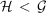of finite groupsbecause the difference of the orders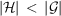does not allow isomorphism. The point groupof a space groupis finite and its order cannot be reduced ifis to be isomorphic to. Therefore, isomorphic subgroups are necessarily k-subgroups.

The number of isomorphic maximal subgroups and thus the number of all isomorphic subgroups of any space group is infinite. It can be shown that maximal subgroups of space groups of index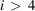are necessarily isomorphic. Depending on the crystallographic equivalence of the coordinate axes, the index of the subgroup is p, p2 or p3, where p is a prime. The isomorphic subgroups cannot be listed individually because of their number, but they can be listed as members of a few series. The series are mostly determined by the index p; the members may be normal subgroups ofor they form conjugacy classes the size of which is either p, p2 or p3. The individual members of a conjugacy class are determined by the locations of their origins. The size of the conjugacy class, a basis for the lattice of the subgroup, the generators of the individual isomorphic subgroups and the coordinate transformation from the coordinate system ofto that ofare listed in IT A1 for all space-group types.

Examples

Isomorphic subgroups of P1: the space group P1 is an abelian space group, all of its subgroups are isomorphic and are normal subgroups. The index may be any prime p.

Isomorphic subgroups of: the space groupis not abelian and subgroups exist of types P1 and. The latter are isomorphic. Those of index 2 are normal subgroups; for higher index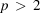they form conjugacy classes of prime size p.

Enantiomorphic space groups have an infinite number of maximal isomorphic subgroups of the same type and an infinite number of maximal isomorphic subgroups of the enantiomorphic type.

Example

All k-subgroupsof a given space group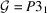with basis vectors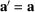,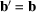,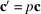, where p is any prime number other than 3, are maximal isomorphic subgroups. They belong to space-group typeif p = 1 mod 3. They belong to the enantiomorphic space-group typeif p = 2 mod 3.

In principle there is no difference in importance between t-, non-isomorphic k- and isomorphic k-subgroups. Roughly speaking, a group–subgroup relation is `strong' if the index [i] of the subgroup is low. All maximal t- and maximal non-isomorphic k-subgroups have indices less than four inand less than five in, index four already being rather exceptional. Maximal isomorphic k-subgroups of arbitrarily high index exist for every space group.